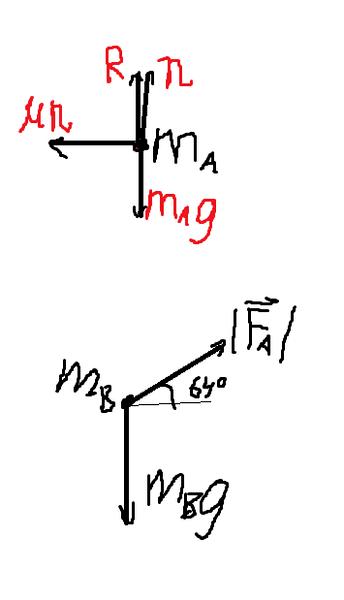# Free body diagram help

A hand supports two books, ma and mb, with ma on top. The hand accelerates the books p and to the right, with a pushing force directed 64° above horizontal. Assuming the upper book does not slip, draw a free body diagram for each book.

My attempt:Which book is on top? and it would help if you defined all of your variables that you have shown.

ma is on top
R is the reaction force
n is the normal force
μn is friction
mg is gravity
|Fa| is the applied force

What force is friction opposing in order to keep it from slipping?

What is your normal force (or contact force)? Is it the same in this situation as it would be if the books were stationary?

What force is friction opposing in order to keep it from slipping?

What is your normal force (or contact force)? Is it the same in this situation as it would be if the books were stationary?
I don't know that's the part I need help with

In this problem friction acts only in the x direction. What other forces do you have acting in x? What do you know about those forces if the book doesnt slip?

It might help to say that the reaction force is the force that the bottom book is pushing up on the top book. So the reaction force is actually the y component of your applied force!

Last edited: